Examples

Chapter 1 Class 10 Real Numbers
Serial order wise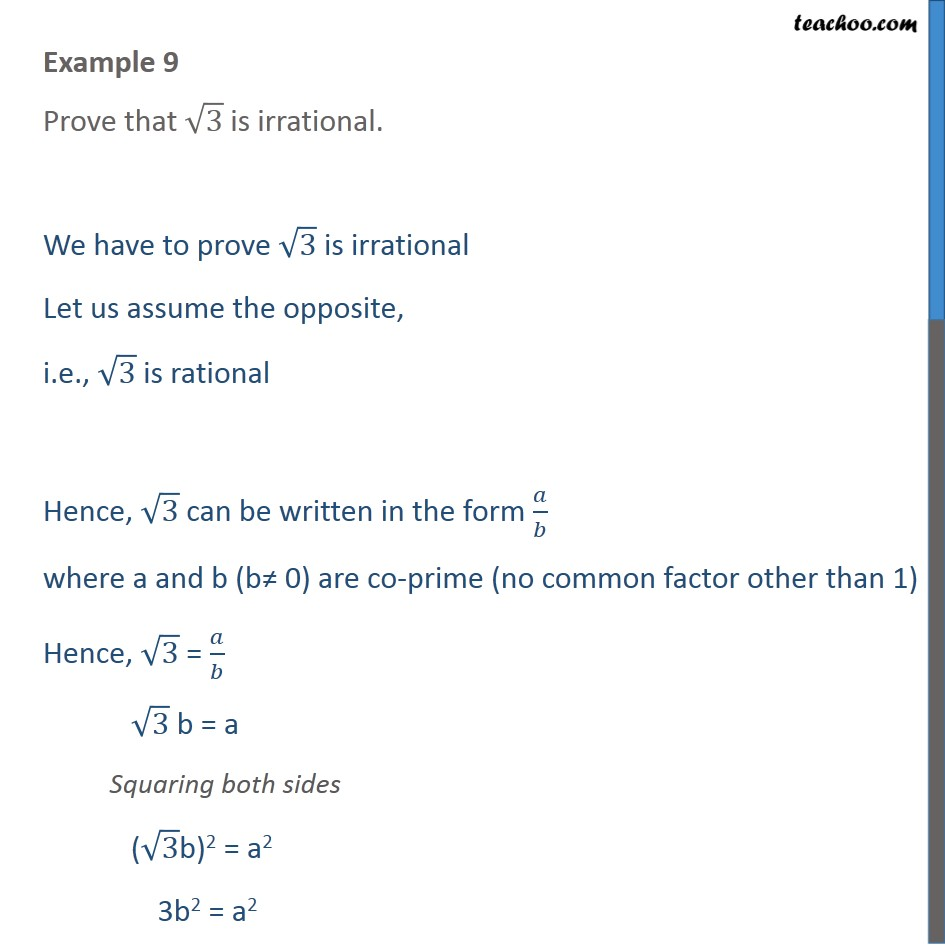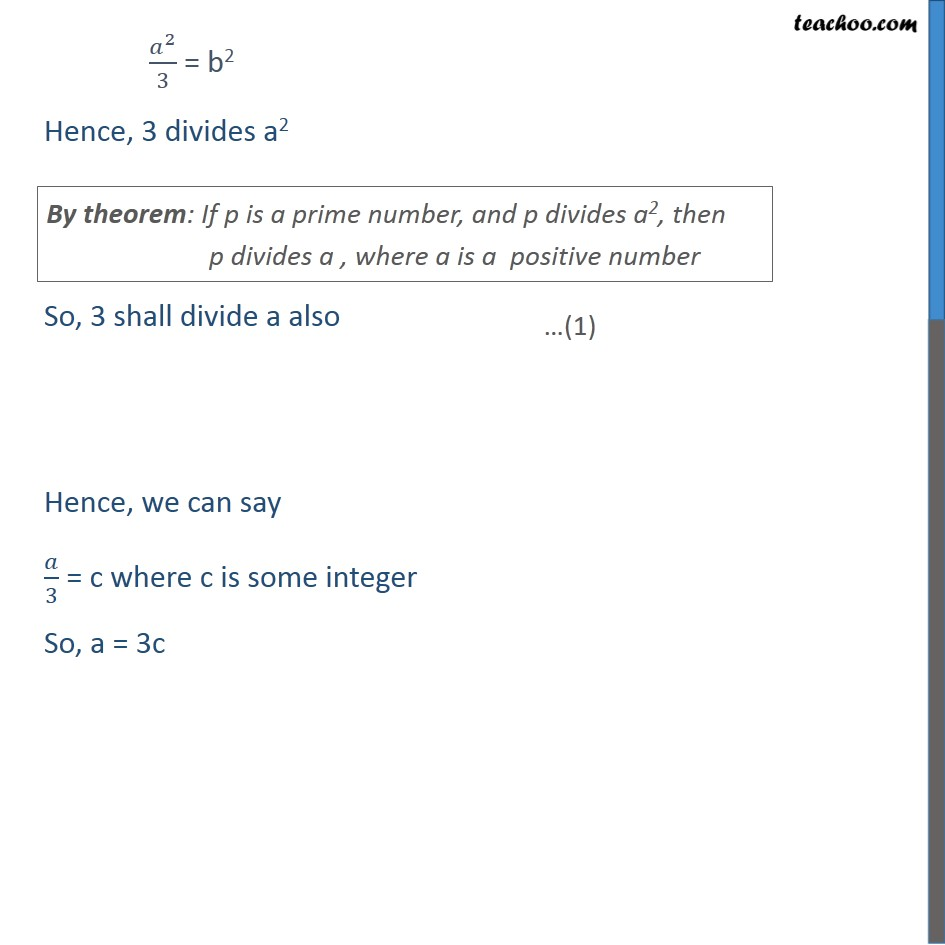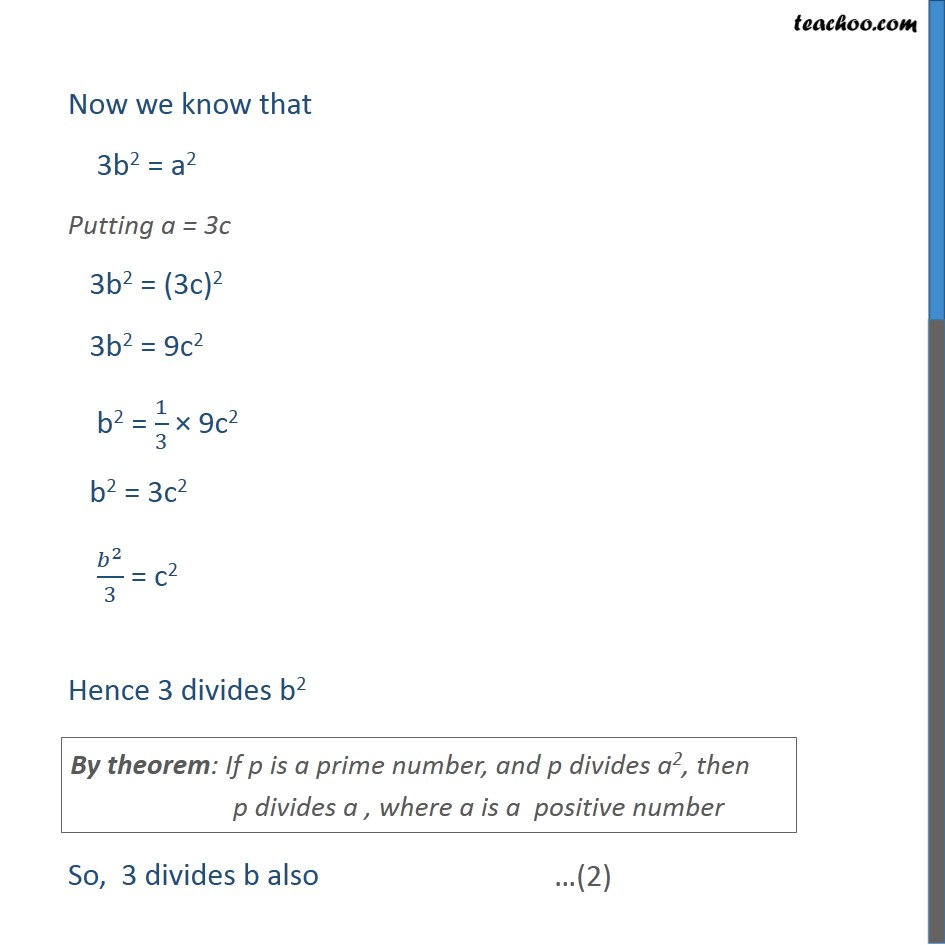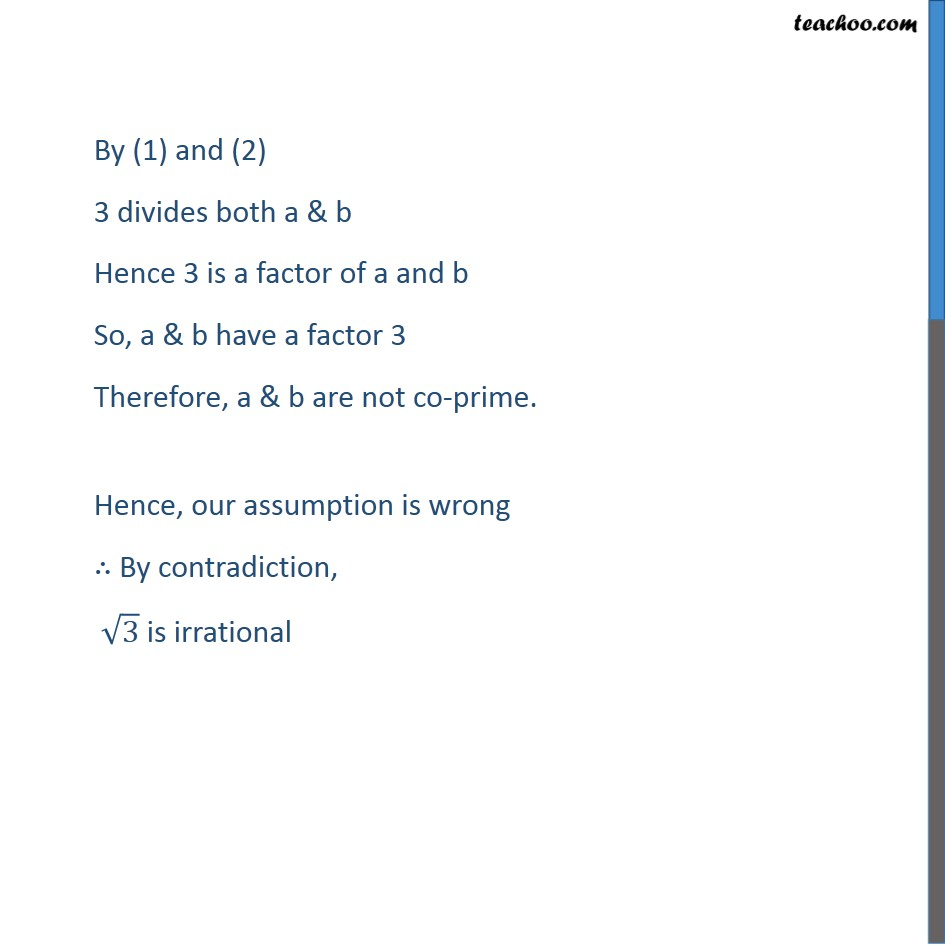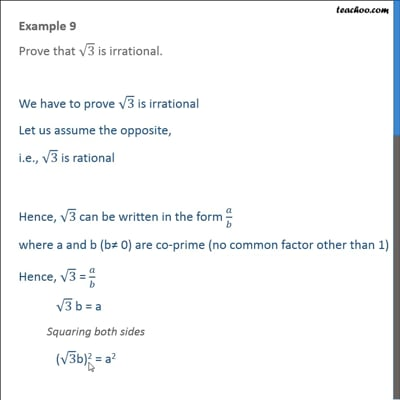This video is only available for Teachoo black users

Solve all your doubts with Teachoo Black (new monthly pack available now!)

### Transcript

Example 9 Prove that 3 is irrational. We have to prove 3 is irrational Let us assume the opposite, i.e., 3 is rational Hence, 3 can be written in the form / where a and b (b 0) are co-prime (no common factor other than 1) Hence, 3 = / 3 b = a Squaring both sides ( 3b)2 = a2 3b2 = a2 ^2/3 = b2 Hence, 3 divides a2 So, 3 shall divide a also Hence, we can say /3 = c where c is some integer So, a = 3c Now we know that 3b2 = a2 Putting a = 3c 3b2 = (3c)2 3b2 = 9c2 b2 = 1/3 9c2 b2 = 3c2 ^2/3 = c2 Hence 3 divides b2 So, 3 divides b also By (1) and (2) 3 divides both a & b Hence 3 is a factor of a and b So, a & b have a factor 3 Therefore, a & b are not co-prime. Hence, our assumption is wrong By contradiction, 3 is irrational